Author: Oscar Cronquist Article last updated on January 19, 2023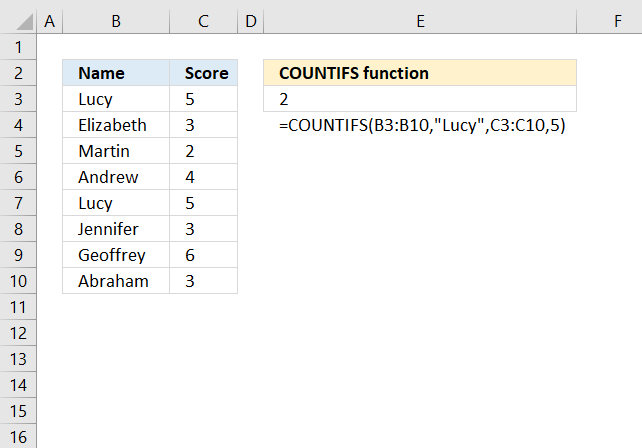The COUNTIFS function calculates the number of cells across multiple ranges that equals all given conditions.

It allows you to use up to 254 arguments or 127 criteria pairs.

## 1. COUNTIFS Function Syntax

COUNTIFS(criteria_range1, criteria1, [criteria_range2, criteria2]…)

## 2. COUNTIFS function Arguments

 criteria_range1 Required. The cell range you want to count the cells meeting a condition. criteria1 Required. The condition that you want to count. [criteria_range2] Optional. Additional ranges, up to 127 pairs. [criteria2] Optional. Additional ranges, up to 127 pairs.

## 3. COUNTIFS function exampleThe COUNTIFS function counts the number of rows that equals one or more conditions.

Formula in cell E3:

=COUNTIFS(B3:B10,"Lucy",C3:C10,5)

Lucy and 5 are found twice, in rows 3 and 7, the COUNTIFS function returns 2 in cell E3. All conditions must be met on the same row.

## 4. COUNTIFS function - Partial match using wildcard characters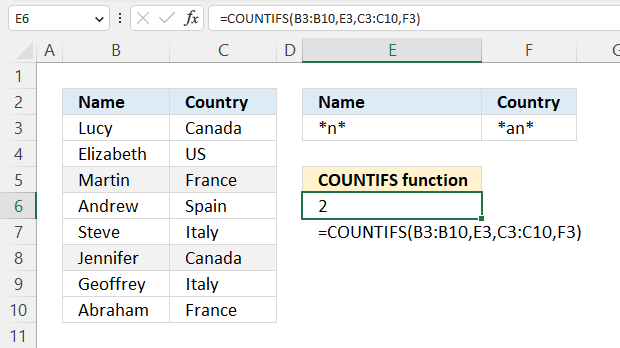The optional wildcard characters make the COUNTIFS function even more powerful, these characters are * asterisk and question marks ? The question mark ? matches a single character while the * asterisk matches any sequence of characters even 0 (zero) characters.

Formula in cell E6:

=COUNTIFS(B3:B10, E3, C3:C10, F3)

### 4.1 Explaining formula

#### Step 1 - Populate arguments

COUNTIFS(criteria_range1, criteria1, [criteria_range2, criteria2]…)

criteria_range1 - B3:B10
criteria1 - E3
criteria_range2 - C3:C10
criteria2 - F3

COUNTIFS(B3:B10, E3, C3:C10, F3)

#### Step 2 - Evaluate COUNTIFS function

COUNTIFS(B3:B10, E3, C3:C10, F3)

becomes

COUNTIFS({"Lucy"; "Elizabeth"; "Martin"; "Andrew"; "Steve"; "Jennifer"; "Geoffrey"; "Abraham"}, "*n*", {"Canada"; "US"; "France"; "Spain"; "Italy"; "Canada"; "Italy"; "France"}, "*an*")

The asterisk matches 0 (zero) to any number of characters, and a leading and trailing asterisk "*n*" matches any value that contains the character n in cells B3:B10. "Martin", "Andrew", "Jennifer" contains a "n"

"*an*" matches any country in cells C3:C10 that contains "an", they are found in rows 3, 5, 8, and 10. The COUNTIFS function counts a row if both conditions are met.

COUNTIFS({"Lucy"; "Elizabeth"; "Martin"; "Andrew"; "Steve"; "Jennifer"; "Geoffrey"; "Abraham"}, "*n*", {"Canada"; "US"; "France"; "Spain"; "Italy"; "Canada"; "Italy"; "France"}, "*an*")

returns

2.

## 5. COUNTIFS function - using two conditions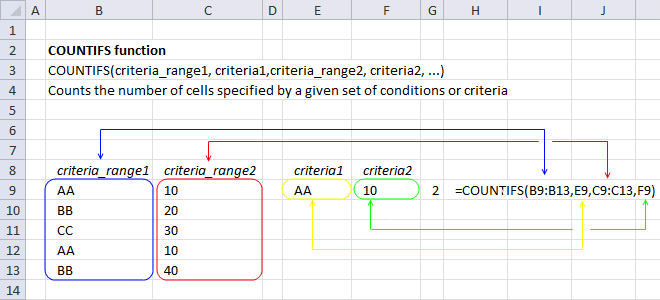This example demonstrates the COUNTIFS function with two conditions applied to two separate columns. The COUNTIFS function counts a record when both conditions are met on the same row.

=COUNTIFS(B9:B13,E9,C9:C13,F9)

This formula checks if text string "AA" is found in cell range B9:B13 and if 10 is found in cell range C9:c13. Row 9 and 12 contain both conditions and 2 are returned to cell G9.

COUNTIFS(B9:B13,E9,C9:C13,F9)

becomes

COUNTIFS({"AA";"BB";"CC";"AA";"BB"},"AA",{10;20;30;10;40},10)

and returns 2 in cell G9.

The calculation above shows two arrays and ou can tell that by the curly brackets {}.

The values in those arrays are separated by a semicolon meaning the values are on a row each.

Here is a post where I use this technique: Highlight duplicate rows

## 6. COUNTIFS function - Logical operators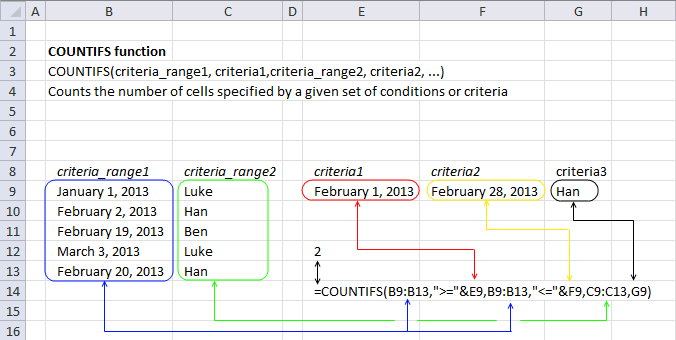The following formula counts how many times text string "Han" equals a cell value in cell range C9:C13, it also checks if dates in cell range B9:B13 are larger than or equal to February 1st, 2013 and smaller than or equal to February 28, 2013.

=COUNTIFS(B9:B13,">="&E9,B9:B13,"<="&F9,C9:C13,G9)

Three conditions in total are applied to two cell ranges, this also demonstrates that you can use logical operators with conditions. Make sure you use double quotes enclosing the logical operators and an ampersand to concatenate the values with the logical operators.

Here is a list of all logical operators you can use and their combinations.

• = equal to
• < less than
• > larger than
• <= less than or equal to
• >=larger than or equal to
• <> not equal to

COUNTIFS(B9:B13, ">="&E9, B9:B13, "<="&F9, C9:C13, G9)

becomes

COUNTIFS({41275; 41307; 41324; 41336; 41325}, ">="&41306, {41275; 41307; 41324; 41336; 41325}, "<="&41333, {"Luke"; "Han"; "Ben"; "Luke"; "Han"}, "Han")

and returns 2 in cell E12. Row 9 and 13 have the word "Han" and are in the month of February 2013.

Here is a post where I use comparison operators: Filter overlapping date ranges

## 7. COUNTIFS function - Count duplicate records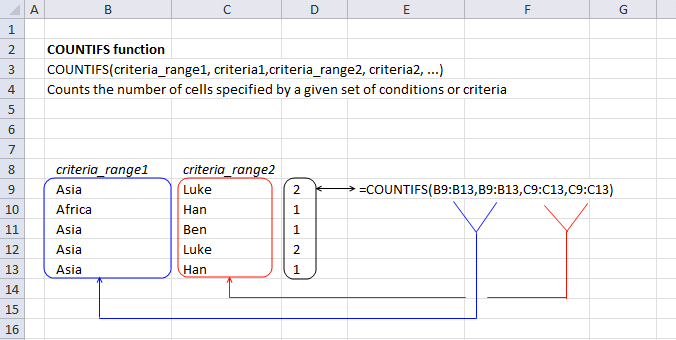The following array formula counts each cell value in each row and returns an array of values.

=COUNTIFS(B9:B13,B9:B13,C9:C13,C9:C13)

I demonstrated in example 1 and 2 above how to use a single condition in each criteria argument, the formula above demonstrates what happens if you use multiple conditions.

Note, you will receive an error if you don't use the same number of conditions in each criteria argument.

COUNTIFS(B9:B13, B9:B13, C9:C13, C9:C13)

becomes

COUNTIFS({"Asia"; "Africa"; "Asia"; "Asia"; "Asia"},{"Asia"; "Africa"; "Asia"; "Asia"; "Asia"},{"Luke"; "Han"; "Ben"; "Luke"; "Han"},{"Luke"; "Han"; "Ben"; "Luke"; "Han"})

and returns this array {2; 1; 1; 2; 1} in cell range D9:D13. This array is interesting because it identifies how many times each record occurs in the data set.

Here are two posts where I use this technique:

## 8. COUNTIFS function - return an array identifying records based on multiple conditions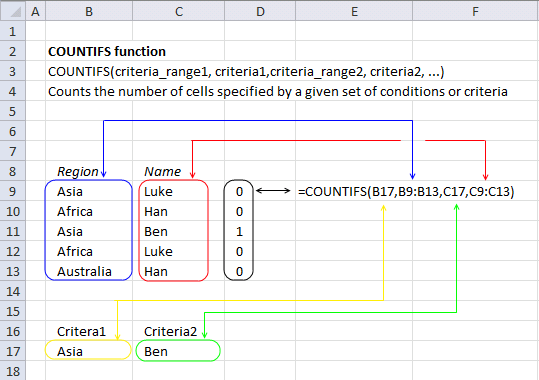In this example, I am using a single cell value as a criteria_range argument and a cell range as criteria argument. This may seem confusing but it is definitely possible and sometimes very useful.

Array formula in cell D9:D13

=COUNTIFS(B17,B9:B13,C17,C9:C13)

### 8.1 How to enter an array formula

Excel 365 users can skip these steps, press Enter to enter the formula like a regular formula.

1. Select cells D9:D13.
2. Type the formula above: =COUNTIFS(B17,B9:B13,C17,C9:C13)
3. Press and hold CTRL + SHIFT simultaneously.
4. Press Enter once.
5. Release all keys.

The formula is now enclosed with curly brackets like this: {=COUNTIFS(B17,B9:B13,C17,C9:C13)}

Don't enter these characters yourself, they appear automatically if you followed the steps above.

### 8.2 Explaining the formula

COUNTIFS(B17, B9:B13, C17, C9:C13)

becomes

COUNTIFS("Asia", {"Asia"; "Africa"; "Asia"; "Africa"; "Australia"}, "Ben", {"Luke"; "Han"; "Ben"; "Luke"; "Han"})

and returns

{0; 0; 1; 0; 0}

in cell range D9:D13.

Row three meets all conditions, 1 is in the third position of the array.

## 9. COUNTIFS function - OR logic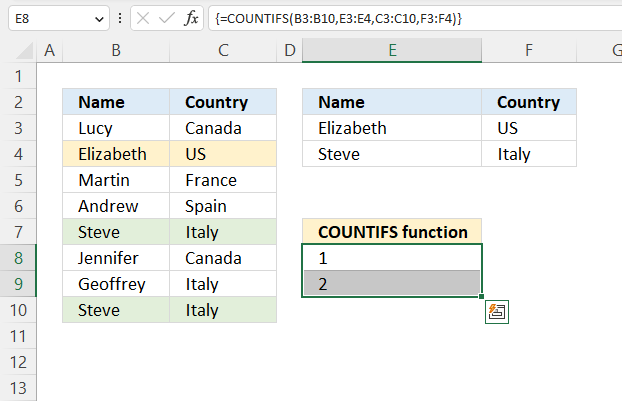The COUNTIFS function performs OR logic between criteria records specified in cells E3:F3 and E4:F4, the way this works is that the array formula calculates both records and returns an array containing numbers that correspond to the position of the records.

Array formula in cells E8:E9:

=COUNTIFS(B3:B10,E3:E4,C3:C10,F3:F4)

### 9.1 How to enter the array formula in cells E8:E9

Excel 365 users can skip these steps, press Enter to enter the formula like a regular formula.

1. Select cells E8:E9.
2. Type the formula above: =COUNTIFS(B3:B10,E3:E4,C3:C10,F3:F4)
3. Press and hold CTRL + SHIFT simultaneously.
4. Press Enter once.
5. Release all keys.

The formula is now enclosed with curly brackets like this: {=COUNTIFS(B3:B10,E3:E4,C3:C10,F3:F4)}

Don't enter these characters yourself, they appear automatically if you followed the steps above.

### 9.2 Explaining formula

#### Step 1 - Populating arguments

COUNTIFS(criteria_range1, criteria1, [criteria_range2, criteria2]…)

becomes

COUNTIFS(B3:B10,E3:E4,C3:C10,F3:F4)

#### Step 2 - Evaluate the COUNTIFS function

COUNTIFS(B3:B10,E3:E4,C3:C10,F3:F4)

becomes

COUNTIFS({"Lucy"; "Elizabeth"; "Martin"; "Andrew"; "Steve"; "Jennifer"; "Geoffrey"; "Steve"},{"Elizabeth"; "Steve"},{"Canada"; "US"; "France"; "Spain"; "Italy"; "Canada"; "Italy"; "Italy"},{"US"; "Italy"})

and returns

{1; 2}.

### 9.3 Sum array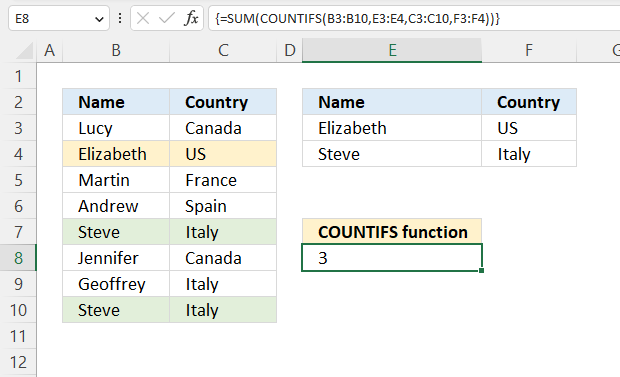This formula adds the numbers for each record and returns a total.

Array formula in cell E8:

=SUM(COUNTIFS(B3:B10,E3:E4,C3:C10,F3:F4))

The SUM function adds the numbers in the array and returns a total.

So if we continue from step 2 above.

SUM(COUNTIFS(B3:B10,E3:E4,C3:C10,F3:F4))

becomes

SUM({1; 2})

and returns 3 in cell E8.

## 10. COUNTIFS function - how to use dates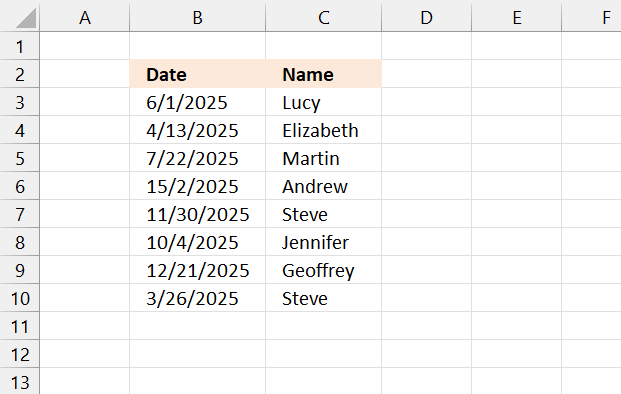First, we need to understand how Excel works with dates. Excel dates are whole numbers formatted as dates. It begins with 1/1/1900 as 1, and 1/1/2000 is 36526.

Here is how to show the numbers behind the dates:

1. Select cell range B3:B10.
2. Press and hold the CTRL key.
3. Press 1. Release the CTRL key. A dialog box appears.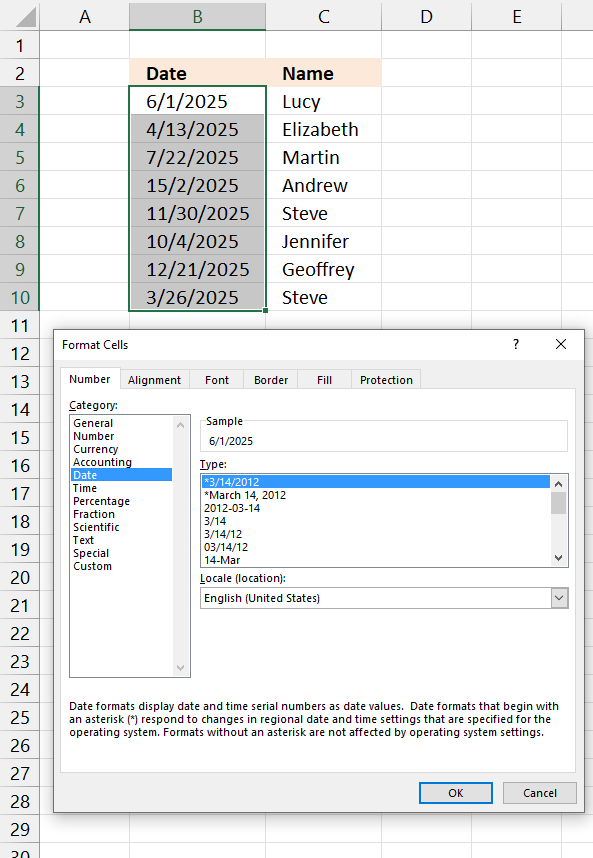4. Select Category: General.5. Press with left mouse button on the OK button.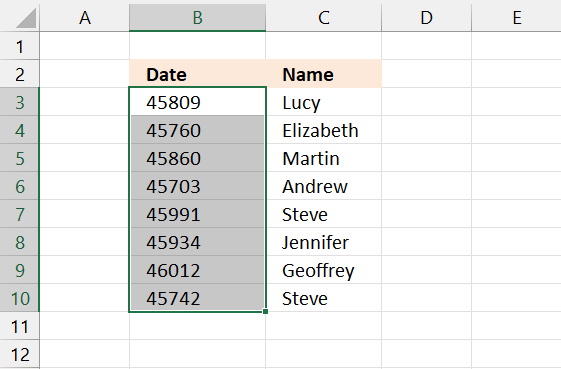6. To go back to dates press and hold the CTRL key, then press z. Release the CTRL key. This keybard shortcut lets you undo the last step.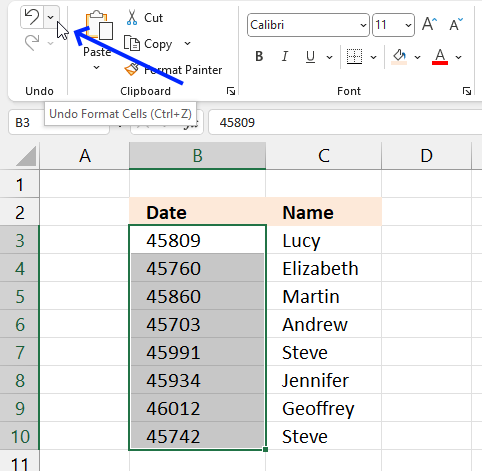Another way to undo the last step is to go to tab "Home" on the ribbon. Press with left mouse button on the "Undo" button, see the image above.We now know that Excel dates are numbers and the COUNTIFS function can easily process numbers.

Use the logical operators in the COUNTIFS function for added functionality.

• < less than sign
• > larger than sign
• = equal sign

## 11. COUNTIFS function - after a date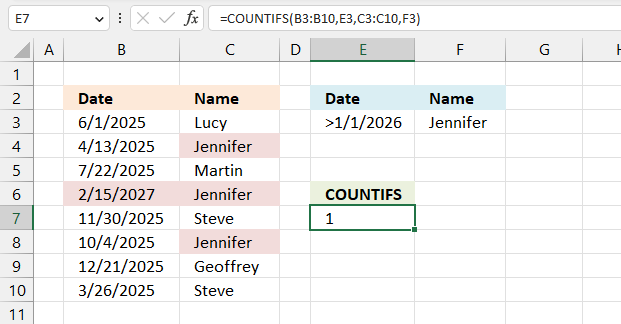This example counts rows that meet two conditions, the first condition is specified in cell F3 (Jennifer). It is matched to the values in column C.

The second condition is specified in cell E3, it is a date condition combined with a logical operator (>1/1/2026). This means that it matches dates later than 1/1/2026.

Only one row meets both condition and that is row 6, the COUNTIFS function returns 1.

Formula in cell E7:

=COUNTIFS(B3:B10,E3,C3:C10,F3)

### Explaining formula

#### Step 1 -  Populate arguments

COUNTIFS(criteria_range1, criteria1, [criteria_range2, criteria2]…)

becomes

COUNTIFS(B3:B10,E3,C3:C10,F3)

#### Step 2 - Evaluate the COUNTIFS function

COUNTIFS(B3:B10, E3, C3:C10, F3)

becomes

COUNTIFS({45809; 45760; 45860; 46433; 45991; 45934; 46012; 45742},">1/1/2026",{"Lucy"; "Jennifer"; "Martin"; "Jennifer"; "Steve"; "Jennifer"; "Geoffrey"; "Steve"},"Jennifer")

and returns 1.

## 12. COUNTIFS function - before a date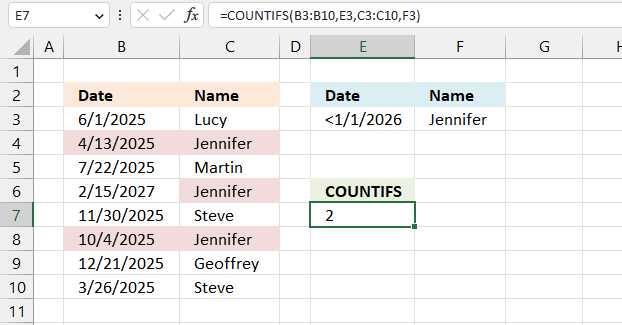This example demonstrates how to count records that equals "Jennifer" in column C specified in cell F3, and the corresponding date in column B is before a given date (1/1/2026) specified in cell E3.

I have highlighted cells that match the criteria, row 6 matches "Jennifer" but not the date condition. Both conditions must be met, however, rows 4 and 8 meet both conditions so the COUNTIFS function returns 2.

Formula in cell E7:

=COUNTIFS(B3:B10,E3,C3:C10,F3)

### Explaining formula

#### Step 1 -  Populate arguments

COUNTIFS(criteria_range1, criteria1, [criteria_range2, criteria2]…)

becomes

COUNTIFS(B3:B10,E3,C3:C10,F3)

#### Step 2 - Evaluate the COUNTIFS function

COUNTIFS(B3:B10, E3, C3:C10, F3)

becomes

COUNTIFS({45809; 45760; 45860; 46433; 45991; 45934; 46012; 45742},"<1/1/2026",{"Lucy"; "Jennifer"; "Martin"; "Jennifer"; "Steve"; "Jennifer"; "Geoffrey"; "Steve"},"Jennifer")

and returns 2.

## 13. How to make the COUNTIFS function work with a dynamic range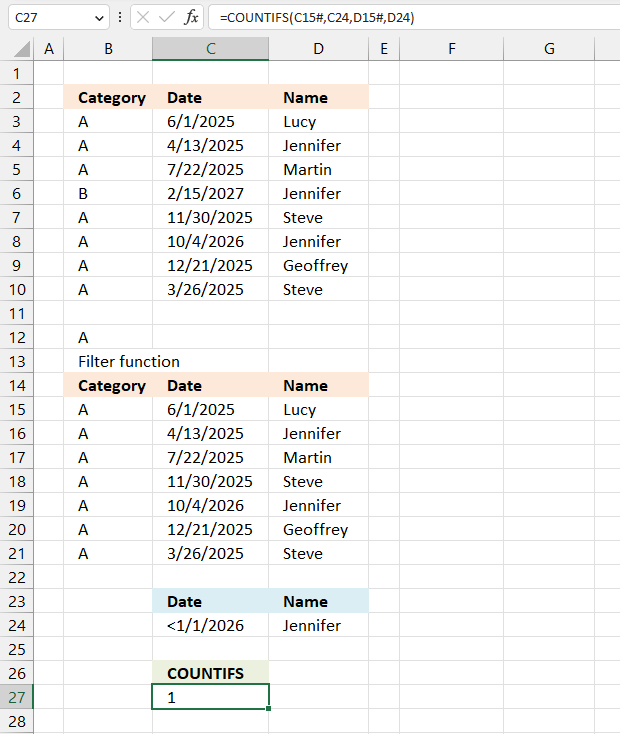There are different types of dynamic ranges:

• Spilled values (Excel 365)
• Excel Table
• Named ranges

This example shows how to reference spilled values in the COUNTIFS function. Spilled values happen when an Excel 365 function returns more than one value, it spills the remaining values below and sometimes to the right as well.

Cell range B15:D15 contains three different FILTER function formulas, they all use the specified value in cell B12 to extract records from cell range B3:D10.

Their results spill to cells below dynamically, by that I mean that if you change the condition in cell B12 their output change and the COUNTIFs function need to adjust to that change dynamically.

Excel 365 formula in cell B15:

=FILTER(B3:B10,B3:B10=B12)

Excel 365 formula in cell C15:

=FILTER(C3:C10,B3:B10=B12)

Excel 365 formula in cell D15:

=FILTER(D3:D10,B3:B10=B12)

COUNTIFS function in cell C27:

=COUNTIFS(C15#, C24, D15#, D24)

The formula above in cell C27 counts spilled rows in B15:D15 based on two conditions specified in cells C24 and D24.

The hashtag lets you reference values dynamically, try to change the condition in cell B12 to "A". The FILTER functions now return only one row, and the COUNTIFS automatically adjusts to the output size.

## 14. How to count entries in Excel by date and an additional condition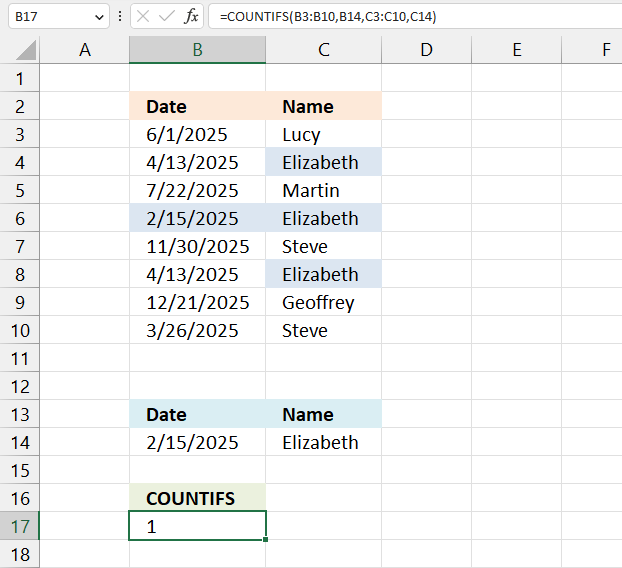This example demonstrates how to use the COUNTIFS function with two conditions, the first one is a date condition and the second is a name condition.

The first condition is specified in cell B14 (Date) and the second condition is in cell C14.

Formula in cell B17:

=COUNTIFS(B3:B10,B14,C3:C10,C14)

### Explaining formula

#### Step 1 -  Populate arguments

COUNTIFS(criteria_range1, criteria1, [criteria_range2, criteria2]…)

becomes

COUNTIFS(B3:B10,B14,C3:C10,C14)

#### Step 2 - Evaluate the COUNTIFS function

COUNTIFS(B3:B10,B14,C3:C10,C14)

becomes

COUNTIFS({45809; 45760; 45860; 45703; 45991; 45760; 46012; 45742},45703,{"Lucy"; "Elizabeth"; "Martin"; "Elizabeth"; "Steve"; "Elizabeth"; "Geoffrey"; "Steve"},"Elizabeth")

and returns 1.